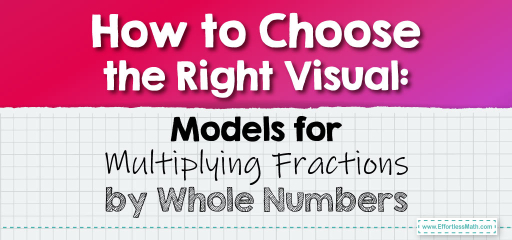# How to Choose the Right Visual: Models for Multiplying Fractions by Whole Numbers

When teaching or learning the multiplication of fractions by whole numbers, visual models can be incredibly helpful. They provide a tangible representation of an abstract concept, making it easier to grasp.But which model should you use? In this guide, we’ll explore different models for multiplying fractions by whole numbers and help you pick the one that’s best suited for your needs.

### Step-by-step Guide:

1. Arrays:

Arrays are grids that can be used to represent fractions. Each row of the grid represents a whole number, and the columns can be shaded to represent fractions.

When to Use: Arrays are great for visual learners and when you want a clear, organized representation of the multiplication process.

2. Number Lines:

Number lines allow for a linear representation of fractions. By marking fractions on the line and making jumps, you can visually see the product.

When to Use: Number lines are ideal when you want to show the progression of multiplication or when dealing with larger whole numbers.

3. Area Models:

Area models use rectangles to represent whole numbers and fractions. The whole number can be represented by the length of the rectangle, and the fraction by its width.

When to Use: Area models are useful when you want to show the spatial relationship between the whole number and the fraction.

4. Set Models:

Set models use groups of objects to represent whole numbers. Each group can then be divided into parts to represent the fraction.

When to Use: Set models are perfect for real-world applications, like when dividing a set of objects (like a bag of candies) into fractional parts.

5. Consider the Context:

The best model often depends on the context in which you’re teaching or learning. For real-world applications, set models might be more intuitive. For pure mathematical understanding, arrays or number lines might be more appropriate.

The Absolute Best Book for 5th Grade Students

### Example 1:

To multiply $$\frac{1}{3}$$ by 3, using:

– Array: Draw 3 rows. Divide each row into 3 parts and shade 1 part in each row. The shaded parts represent the product.

– Number Line: Mark $$\frac{1}{3}$$ on the line and make 3 jumps of this size. The endpoint represents the product.

### Example 2:

To multiply $$\frac{2}{5}$$ by 4, using:

– Area Model: Draw a rectangle with length 4. Divide the width into 5 equal parts and shade 2 of them. The shaded area represents the product.

– Set Model: Imagine 4 bags of candies. If each bag is divided into 5 equal parts, and you take 2 parts from each, you’ve taken the product of $$\frac{2}{5}$$ and 4.

### Practice Questions:

1. Multiply $$\frac{1}{4}$$ by 5 using an array.

2. Multiply $$\frac{3}{6}$$ by 2 using a number line.

3. Multiply $$\frac{2}{7}$$ by 3 using an area model.

A Perfect Book for Grade 5 Math Word Problems!

1. Draw 5 rows, divide each into 4 parts, and shade 1 part in each row. The product is $$\frac{5}{4}$$ or $$1 \frac{1}{4}$$.

2. Mark $$\frac{3}{6}$$ or $$\frac{1}{2}$$ on the line and make 2 jumps of this size. The endpoint represents 1.

3. Draw a rectangle with length 3. Divide the width into 7 equal parts and shade 2 of them. The shaded area represents $$\frac{6}{7}$$.

The Best Math Books for Elementary Students

### What people say about "How to Choose the Right Visual: Models for Multiplying Fractions by Whole Numbers - Effortless Math: We Help Students Learn to LOVE Mathematics"?

No one replied yet.

X
51% OFF

Limited time only!

Save Over 51%

SAVE $15 It was$29.99 now it is \$14.99